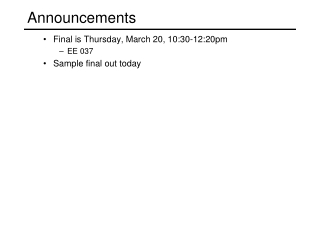DownloadDownload PresentationAnnouncements

# Announcements

Download Presentation## Announcements

- - - - - - - - - - - - - - - - - - - - - - - - - - - E N D - - - - - - - - - - - - - - - - - - - - - - - - - - -
##### Presentation Transcript

1. Announcements • Final is Thursday, March 20, 10:30-12:20pm • EE 037 • Sample final out today

2. Filtering • An image as a function • Digital vs. continuous images • Image transformation: range vs. domain • Types of noise • Noise reduction by averaging multiple images • Cross-correlation and convolution • properties • mean, Gaussian, bilinear filters • Median filtering • Image scaling • Image resampling • Aliasing • Gaussian pyramids

3. Edge detection • What is an edge and where does it come from • Edge detection by differentiation • Image gradients • continuous and discrete • filters (e.g., Sobel operator) • Effects of noise on gradients • Derivative theorem of convolution • Derivative of Gaussian (DoG) operator • Laplacian operator • Laplacian of Gaussian (LoG) • Canny edge detector (basic idea) • Effects of varying sigma parameter • Approximating an LoG by subtraction

4. Features • What makes a good feature? • Derivation in terms of shifting a window • H matrix • Definition • Meaning of eigenvalues and eigenvectors • Harris operator • How to use it to detect features • Feature descriptors • MOPS (rotated square window) • SIFT (high level idea) • Invariance (how to achieve it) • Rotation • Scale • Lighting • Matching features • Ratio test • RANSAC

5. Projection • Properties of a pinhole camera • effects of aperture size • Properties of lens-based cameras • focal point, optical center, aperture • thin lens equation • depth of field • circle of confusion • Modeling projection • homogeneous coordinates • projection matrix and its elements • types of projections (orthographic, perspective) • Camera parameters • intrinsics, extrinsics • types of distortion and how to model

6. Mosaics • Image alignment • Image reprojection • homographies • spherical projection • Creating spherical panoramas • Handling drift • Image blending • Image warping • forward warping • inverse warping

7. Projective geometry • Homogeneous coordinates and their geometric intuition • Homographies • Points and lines in projective space • projective operations: line intersection, line containing two points • ideal points and lines (at infinity) • Vanishing points and lines and how to compute them • Single view measurement • computing height • Cross ratio • Camera calibration • using vanishing points • direct linear method

8. Stereo • Epipolar lines • Stereo image rectification (basic idea) • Stereo matching • window-based epipolar search • effect of window size • sources of error • Energy-minimization (MRF) stereo (basic idea) • Depth from disparity • Active stereo (basic idea) • structured light • laser scanning

9. Structure from motion • Correcting drift in mosaics through global optimization • Least squares • Structure from motion • Solving for camera rotations, translations, and 3D points • The objective function • The pipeline (from Photo tourism slides: detection, matching, iterative reconstruction…) • Photo tourism

10. Light, perception, and reflection • Light field, plenoptic function • Light as EMR spectrum • Perception • color constancy, color contrast • adaptation • the retina: rods, cones (S, M, L), fovea • what is color • response function, filters the spectrum • metamers • Finding camera response function (basic idea, not details) • Materials and reflection • what happens when light hits a surface • BRDF • diffuse (Lambertian) reflection • specular reflection • Phong reflection model • measuring the BRDF (basic idea)

11. Photometric stereo • Shape from shading (equations) • Diffuse photometric stereo • derivation • equations • solving for albedo, normals • depths from normals • Handling shadows • Computing light source directions from a shiny ball • Limitations

12. Recognition • Classifiers • Probabilistic classification • decision boundaries • learning PDF’s from training images • Bayes law • Maximum likelihood • MAP • Principle component analysis • Eigenfaces algorithm • use for face recognition • use for face detection

13. Segmentation • Graph representation of an image • Intelligent scissors method • Image histogram • K-means clustering • Morphological operations • dilation, erosion, closing, opening • Normalized cuts method (basic idea)

14. Motion • Optical flow problem definition • Aperture problem and how it arises • Assumptions • Brightness constancy, small motion, smoothness • Derivation of optical flow constraint equation • Lucas-Kanade equation • Derivation • Conditions for solvability • Relation to Harris operator • Iterative refinement • Newton’s method • Pyramid-based flow estimation

15. Texture • Markov chains • Text synthesis algorithm • Markov random field (MRF) • Efros and Leung’s texture synthesis algorithm • Improvements • Fill order • Block-based • Texture transfer (basic idea)

16. Guest Lectures • Richard Ladner—Tactile graphics • Jenny Yuen—cateract detection • Jeff Bigham—object-based image retrieval • (basic ideas)